[Contents]

Single- and Multi-Variable Linear Least Squares
John Denker

## 1  Introduction

Linear least squares is also known as linear regression. It is used for fitting a theoretical curve (aka model curve, aka fitted function) to a set of data.

Fun fact #1: The word “linear” in this context does mean that the fitted function is a straight line (although it could be). It could perfectly well be a polynomial, where the monomials are nonlinear, or it could be a Fourier series or some such, where the basis functions are highly nonlinear. The key requirement is that the coefficients i.e. the parameters that you are adjusting must enter the fitted function linearly.

Linear regression is by no means the most general or most sophisticated type of data analysis, but it is widely used because it is easy to understand and easy to carry out.

## 2  A Simple Example : Determining Density

Let’s start with a simple example. (A less-simple more-realistic example is discussed in section 4. Some of the principles involved, and some additional examples, are presented in section 5.)

Suppose we want to compute the density of some material, based on measuring three samples. The volume and mass of the samples are shown in table 1.

 V M Volume Mass 1.000 1.062 1.100 1.091 1.200 1.211
Table 1: Volume and Mass – Raw Data

This is Monte Carlo data, which is a fancy way of saying it was cooked up with the help of the random-number generator on a computer. The volume readings are evenly spaced. The mass readings are drawn from a random distribution, based on a density of ρ=1 (exactly) plus Gaussian random noise with a standard deviation of 0.05. All the data and plots in this section were prepared using the spreadsheet in reference 1.

1.    In accordance with standard good practice, the first thing to do is to plot the data and look at it, to see whether it makes sense. This is shown in figure 1. When we look at this, nothing jumps out at us, which is OK.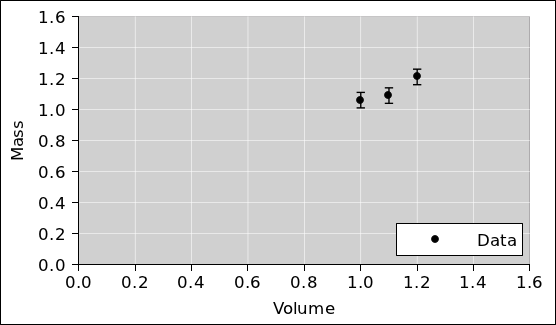Figure 1: Mass Versus Volume : Raw Data

2.    The obvious next step is to calculate the density. We do this for each sample, on a sample-by-sample basis, as shown in table 2.

 V M ρ Volume Mass Density 1.000 1.062 1.062 1.100 1.091 0.992 1.200 1.211 1.010 Naïve Average 1.021
Table 2: Volume, Mass, and Calculated Density

We plot this data as well, and look at it.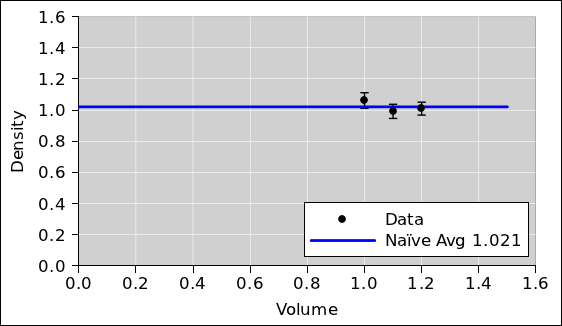Figure 2: Density Versus Volume

We can estimate the average density graphically, by hand, with the help of a transparent ruler. Draw a horizontal line so that the data points are distributed symmetrically above and below the line. Note:

• It is important that the ruler be transparent. Otherwise you will introduce bias, typically by under-emphasizing the data points that are not visible.
• It is important to keep the line horizontal. Zero slope. This is easier to do if you plot the data on graph paper (as opposed to unadorned typing paper).

Optionally, we can take the average of these three densities numerically, as shown at the bottom of table 2, although this is slightly naïve (for reasons discussed in item 3). The value we compute is very nearly the same as the value corresponding to the height of the horizontal line we drew using the transparent ruler, which makes sense. This is plotted in figure 2.

Note that taking the average is tantamount to a one-parameter fit. There is only one quantity to be determined from the data, namely the average density.

3.    We can perform a less-naïve analysis if we realize that the mass data has uniform error bars but the density data does not. That’s because when we divide the mass by the volume, the error bars get divided also. Therefore we should perform a weighted average, giving the larger samples larger emphasis, in proportion to the inverse square of the error bars.

 V M ρ Weight Volume Mass Density Factor 1.000 1.062 ±.05 1.062 ±.05 1.000 1.100 1.091 ±.05 0.992 ±.046 1.210 1.200 1.211 ±.05 1.010 ±.042 1.440 Naïve Weighted Average Average 1.021 1.018
Table 3: Volume, Mass, and Weighted-Average Density

Again, we plot the data and look at it. Again we note that taking the average is tantamount to fitting the data using a one-parameter model i.e. a zeroth-order polynomial i.e. a horizontal straight line.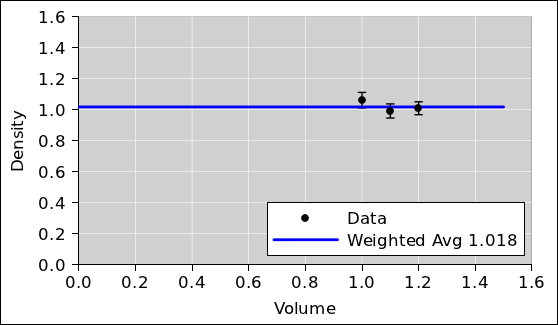Figure 3: Density Data plus Weighted Average

4.    It is possible to analyze the raw mass-versus-volume data directly, using it in the form we see in figure 1, without explicitly calculating the density of each sample. This is a tricky approach. It works fine if done properly, but we have to be careful.

The key step in the analysis is to draw a straight line through the data, as shown in figure 4.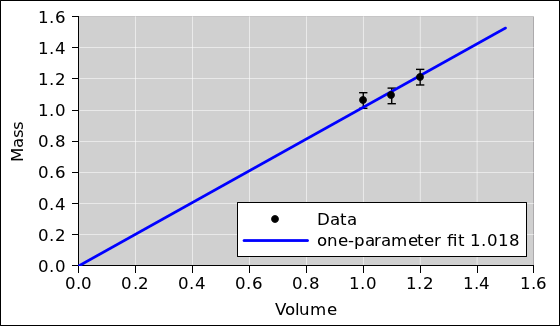Figure 4: Mass versus Volume plus Fitted Line

This can be done by hand using a transparent ruler. Draw a line so that the data points are distributed symmetrically above and below the line. Note:

• As mentioned in item 2, it is important that the ruler be transparent.
• It is important to pin the ruler so that the fitted line goes through the origin. We know for sure that the graph of mass versus density must do this, in accordance with equation 1b. We know this absolutely, a priori, as a consequence of the official definition of density, equation 1a.

 ρ := M / V (1a) M = ρ V (1b)

Pinning the ruler gives us a one-parameter model. In fact if we do it properly, it gives the same result as the weighted average in item 3 – the same conceptually, numerically, and in every other way. It should come as no surprise that we want a one-parameter model, given that we used one-parameter models in item 2 and item 3. See section 3.1 for more about how and why we pin the ruler.

Refer to section 3.2 and section 3.3, if you dare, for a discussion of some of the misconceptions that can arise in conjunction with this approach.

5.    We can also use a computer to calculate the best-fit straight line directly. Specifically, we can use the linest(y_vec, basis_vecs) spreadsheet function. The result is identical to what we see in figure 4. The slope found by linest(...) is exactly equal to the weighted-average density we calculated in table 3, as it should be.

6.    When using a computer – just as when using a transparent ruler – it is important to constrain the fit so that the mass-versus-volume curve goes through the origin. This can be arranged by setting to zero the optional third argument to the linest(...) function. Keep in mind that equation 1b is of the form y=mx which has one adjustable parameter (the slope). It is emphatically not of the form y=mx+b which would have two adjustable parameters (the slope and the y-intercept). When linest(...) peforms the fit, we need it to perform a one-parameter fit, not a two-parameter fit.

If you don’t believe me, take a look at figure 5. The red line shows what would happen if you performed a two-parameter straight-line fit. It’s a horror show. The slope of the two-parameter fit is wildly different from the actual density. The one-parameter fit (shown in blue) does a much, much better job.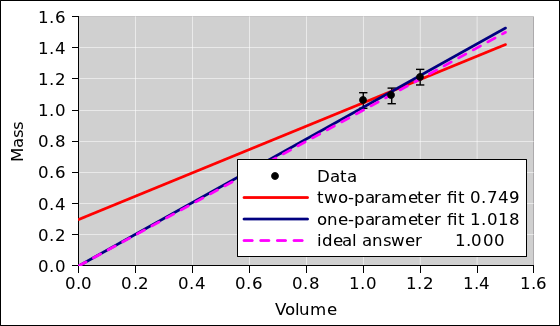Figure 5: One-Parameter versus Two-Parameter Fit

Keep in mind that the details depend on how much data we have, and now noisy it is. If we had 300 data points rather than 3 data points, we would be better able to afford adding fitting parameter, but even then we would need some specific rationale for doing so.

## 3  Discussion

### 3.1  How and Why We Pin the Ruler

In connection with figure 5, when we speak of pinning the ruler, you could use an actual push-pin (with a suitable backing pad), but the usual good practice is to use your pencil or pen. Hold the tip of your pen at (0, 0). Gently push the ruler up against it, and use it as a pivot. In other words, use your pen as a pin. This trick is widely used by artists, wood-shop and metal-shop workers, draftsmen, et cetera.

Note that the situation shown in figure 5 is very common and utterly typical. The red line illustrates an important general principle, namely that it is a very bad idea to use “extra” adjustable parameters when fitting your data. This principle is known as Occam’s Razor and has been known for more than 700 years.1 Narrowly speaking, the red line does a better of fitting the data; it just does a much worse job of explaining and modeling the data. That is to say, the red line actually comes closer to the data points than the blue line does. However, our goal is to interpret the data in terms of density, and the red line does not help us with that. Equation 1b provides us with a sensible model of the situation, and the blue line implements this model. The slope of the blue line can be directly interpreted as density.

Some descriptive terminology:

• In the statistics literature, this situation is sometimes referred to as a bias-variance tradeoff.
• If you use too many parameters, you wind up fitting the noise (rather than fitting the signal).
• Similarly, using too many parameters is known as overfitting the data.

For a simple yet modern introduction to the fundamental issues involved, see reference 2. See section 3.3 for more about the perils of superfluous parameters. If not pinned, the ruler implements at two-parameter model. Just because you could use a two-parameter model doesn’t mean you should. Just because the tool allows a two-parameter fit doesn’t mean it is a good idea. As the ancient proverb says,:

 It is a poor workman who blames his tools.

### 3.2  How Not to Pin the Ruler

This section exists only to deal with a misconception. If you don’t suffer from this particular misconception, you are encouraged to skip this section.

Some people try to explain pinning the ruler by saying we are fitting the curve to an imaginary data point at (0, 0) ... but I do not recommend this way of thinking about it. It is better to base the decision on good, solid theory than on imaginary data. We do indeed have a good, solid theory, namely the definition of density, equation 1a.

In some sense it would be the easiest thing in the world to take data at (0, 0). We know exactly how much a zero-sized sample weighs, and we know exactly how much volume a zero-sized sample displaces. So this data point is in some sense more accurate than any of the data you see in table 3. However, this data point would be hard to analyze using the method of table 3, because we cannot divide zero mass by zero volume to get information about the density. This is harmless but uninformative. It just reflects the fact that for any density whatsoever, the mass-versus-volume curve must pass through (0, 0).

It must be emphasized that all the calculations involved in table 3 can be and should be done without reference to any imaginary data at the origin ... or any real data at the origin. In third grade you learned how to divide one number by another, and that is all that is needed to calculate the density in accordance with equation 1a.

Starting from

ρ :=
 M V
(2)

you can, if you want, rewrite this so that it looks like the slope of a line:

ρ =
 M − 0 V − 0
(3a)
=
 ΔM ΔV
(3b)
= slope from (0,0) to (V,M) ???     (3c)

You are free to do this, but you are not required to. Furthermore, the legitimacy of the step from equation 2 to equation 3a is guaranteed by the axioms of arithmetic. It does not depend on any fake data (or real data) at the origin.

Let’s be clear: I do not want to hear any complaints about “fake” data at the origin, for multiple reasons:

1. There is no need to use any data at the origin, fake or otherwise. There is no need to even mention the origin. You learned in third grade how to divide one number by another, and that is all that is required. You are not obliged to interpret the density in terms of the slope of a line. If you want to make this dubious interpretation as shown in equation 3c, do so at your own risk.
2. Consider the fitting procedure: Data goes in, and a fitted model comes out. No matter whether or not you use any (0, 0) data as input, the fitted mass-versus-volume curve that comes out will pass through the origin, assuming we do things properly. This is guaranteed by the definition of density.
3. If you wanted to take some non-fake data at the origin, or infinitesimally close to the origin, you could do so.
4. If you create an artificial problem, you ought not brag if you manage to solve it. If you create an unnecessary problem, you ought not whine if you fail to solve it. Using a two-parameter model of the form y=mx+b for the density is an artificial, unnecessary problem of just this sort. You can partially alleviate or conceal the damage by using fake data (or real data) at or near the origin to constrain the extra parameter at the only reasonable value, namely b=0. You ought not brag about or whine about this partial success. The fundamental point is that you should have been using a one-parameter model all along.

The whole idea of using a two-parameter model in mass-versus-volume space is sophomoric, i.e. pseudo-sophisticated yet dumb at the same time. The normal approach would be to calculate the densities and then average them in the obvious way, as in figure 3. People get into trouble if they are sophisticated enough to realize that they can analyze the data in mass-versus-volume space, but not sophisticated enough to do it correctly.

### 3.3  How Not to Justify Superfluous Parameters

This is another section that exists only to deal with a misconception. If you don’t suffer from this particular misconception, you are encouraged to skip this section.

Sometimes people who ought to know better suggest that “extra” parameters are a way of detecting and/or dealing with systematic errors. This is completely wrong, for multiple reasons.

Example #1: Let’s consider the scenario where Alice and Bob are lab partners. Alice takes some of the data, and Bob takes the rest. Alice screws up the tare, but Bob doesn’t. The data is shown in figure 6. Specifically, the two-parameter straight-line fit to the data, as shown by the red line, has a negligible y-intercept. The slope, meanwhile, is significantly wrong i.e. not equal to the actual density.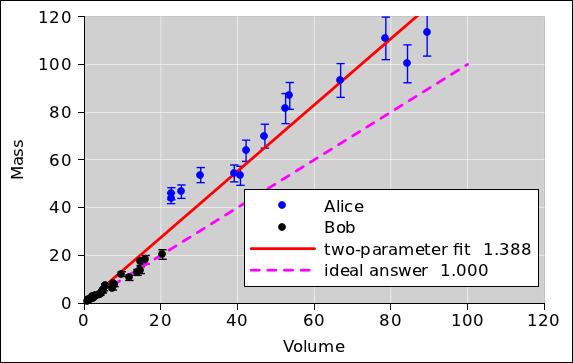Figure 6: Peculiar Systematic Error

It should be obvious that in this scenario, allowing for a nonzero y-intercept is nowhere near sufficient to detect the problem, let alone resolve it.

In contrast, plotting the data and looking at it, as suggested in item 1, helps a lot.

To repeat: You should never increase the number of parameters in your model without a good reason. Mumbling vague words about some unexplained “experimental error” is never a sufficient rationale. That’s the sort of thing that leads to the disaster shown by the red curve in figure 5 and/or figure 6.

Example #2: If you systematically use the wrong units, e.g. CGS units (g/cc) instead of SI units (kg/m3), there result will suffer from a huge systematic error, but fitting the data using a superfluous y-intercept will be completely ineffective at detecting the problem, let alone resolving it.

Example #3: Porosity can cause systematic errors in any determination of density. These cannot be reliably detected, let alone corrected, by throwing in an “extra” parameter without understanding what’s going on. See section 4.

Example #4: If you forgot to tare the balance in a way that affected all the data equally, it would lead to a nonzero y-intercept on the pseudo-mass-versus-volume curve. However, the converse is not true. The huge y-intercept on the red curve in figure 5 is absolutely not evidence of a screwed-up tare. Conversely, the absence of a significant y-intercept in figure 6 is absolutely not evidence of the absence of systematic error. Furthermore, even if the data behind figure 5 included a screwed-up tare, using a two-parameter fit would not be an appropriate way to resolve the problem. It would just add to your problems. You would have all the problems associated with the red curve in figure 5, plus a screwed-up tare. The correct procedure would be to go back and determine the tare by direct measurement. If this requires retaking all three data points, so be it.

The red curve in figure 6 shows what happens if you try to detect and/or deal with a problem without fully understanding it. Again, the best procedure is to do things right the first time. In an emergency, if you wanted to correct the mistake in figure 6, you would first need to understand the nature of the mistake, and then cobble up a complicated model to account for it. Just throwing in an adjustable y-intercept and hoping for the best is somewhere between unprofessional and completely insane.

If you have a very specific, well-understood nonideality in your experiment, the best procedure is to redesign the experiment to remove the nonideality. The second choice would be to make a richer set of measurements, so that you get independent observations of whatever is causing the nonideality ... for instance by making independent observations of the tare. Failing that, if you are sure you understand the nonideality, it might be OK to complexify the model so that it accounts for that specific nonideality, such as the fancy stepwise correction we see in the cyan curve in figure 7. We use a model of the form y=mx for Bob’s data and a model of the form y=mx+b for Alice’s data. Note that m is the same for both, as it should be, since we are trying to determine the density and it should be the same for both. In this scenario we have an order of magnitude more data than we did in section 2 41 points instead of 3 points – so the additional parameter (b) does not cause nearly so much trouble. Away from the step, the slope (m) of the cyan curve is constant and is a very good estimate of the density we are trying to measure. However, even in the best of circumstances, adding variables comes at a cost. You need to make sure you have enough data points (and short enough error bars) so that you can successfully ascertain values for all the parameters. That is, you need to make sure you are on the right side of the bias/variance tradeoff.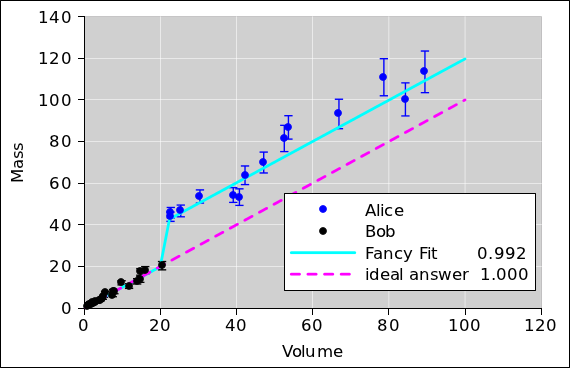Figure 7: Fancy Correction for Well-Understood Error

### 3.4  Maximum Likelihood, Or Not

Keep in mind that all the methods discussed in this document are least-squares methods. That means they are in the category of maximum likelihood methods. In other words, they calculate the conditional probability of the data, given the model. For virtually all data-analysis purposes, that’s the wrong way around. What you really want is the probability of the model, given the data. The details of how to do this right are beyond the scope of this document. For simple tasks such as the density determination in section 2 you can get away with maximum likelihood, but please do not imagine that there is any 11th commandment that says maximum likelihood is the right thing to do. A lot of people who ought to know better assume it is OK, even when it isn’t.

### 3.5  Data Analysis : Closing the Loop

Whenever you need to analyze data, you should test your methods. The procedure outlined in section 2 is a good empirical check (but not the only possible check).

Starting from a set of reasonable parameters, generate some artificial data. Add some Monte Carlo noise to the data. Then analyze the data and see how accurately you get the right answer, i.e. how closely the fitted parameters agree with the parameters you started with.

This kind of check is called “closing the loop” around the data reduction process. The closed loop is shown in figure 8. Closing the loop is considered standard good practice aka due diligence.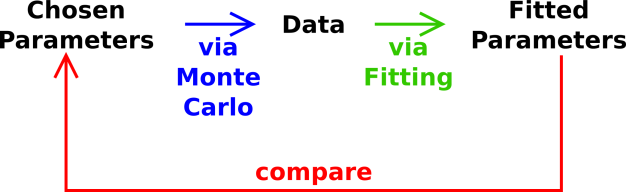Figure 8: Closing the Loop around the Analysis

### 3.6  More General Regression Curves

The linest(...) spreadsheet function is often used for finding a “trend line” ... but it is capable of doing much more than that. In general, it finds a regression curve, which not be a straight line.

• It can find the coefficients of the best-fit quadratic. An example of this is given in section 5.7.
• It can also handle higher-order polynomials.
• It can find the coefficients of the best-fit Fourier series. An example of this is given in section 5.8.
• More generally, it can fit to an arbitrary linear combination of basis functions, using whatever basis functions you choose.

In statistics, this fitting procedure is called linear regression. It must be emphasized that this method requires the fitted function (the regression curve) be a linear combination of the basis functions, but the basis functions themselves do not need to be linear functions of X (whatever X may be).

## 4  Density, Again

Let’s do another density determination. This time the task will be somewhat more realistic and more challenging. We will have to apply a higher degree of professionalism. The spreadsheet used to construct the model and do the analysis is available; see reference 1.

In the real world, it is usually easier to measure mass accurately than to measure volume accurately. Therefore, in this section we have arranged for the artificial data to have significant error bars in the volume direction but not in the mass direction, as you can see in figure 9.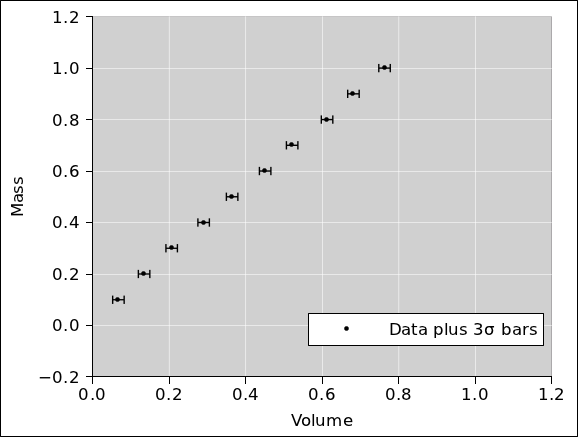Figure 9: Mass versus Volume : Raw Data

In a logical world, data-analysis procedures would be able to handle uncertain abscissas just as easily as uncertain ordinates. However, the most commoly-available procedures are not as logical as you might have hoped. They do not handle uncertain abscissas at all well. Therefore in figure 10 we replot the data using mass as the abscissa. It must be emphasized that figure 9 and figure 10 are two ways of representing exactly the same data. (For clarity, the error bars in these two figures are 3σ long. All other figures use ordinary 1σ bars.)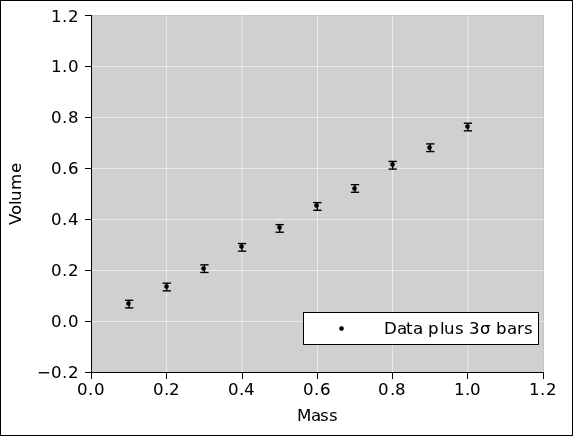Figure 10: Volume versus Mass : Raw Data

We divide mass by volume to get the density. This is done numerically, on a sample-by-sample basis, just as we did in section 2. The numerical data table can be found in reference 1. The results are plotted in figure 11. The naïve (unweighted) average is also shown.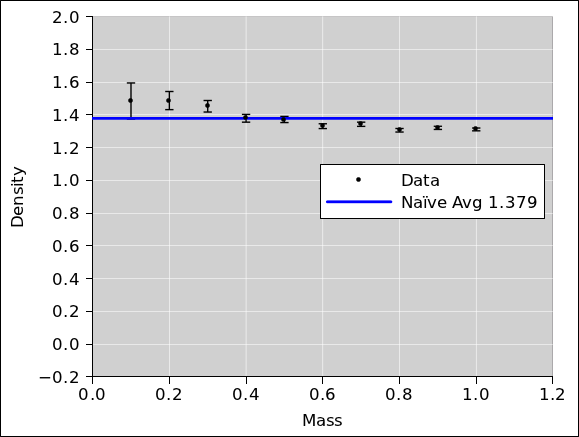Figure 11: Density versus Mass

At first glance, the data doesn’t look too terrible. However, if you look more closely, it looks like there might be something fishy going on. The low-mass data might be systematically high, while the high-mass data might be systematically low.

It’s hard to tell what’s going on just by looking at the data in this way. The professional approach is to look at the residuals. That is, we subtract the fitted average from the data and see what’s left. Even better, if possible, we normalize each of the residuals by its own error bar. Then, to make the data fit nicely on the graph, we reduce the residuals by a factor of 10. This is shown in figure 12. The properly-weighted average is also shown.

Note that normalizing the residuals is easy for artificial data but may be more difficult for real-world data. At this stage in the analysis, you might or might not have a good estimate for the error bars on the data. If necessary, make an order-of-magnitude guess and then forge ahead with the preliminary analysis. With any luck, you can use the results of the preliminary analysis to obtain a reasonable estimate of the uncertainy. You can then re-do the analysis using the estimated uncertainty, and check to see whether everything is consistent.

By the same token, it is pointless to quote the chi-square of a fit, if you are depending on the fit to obtain an estimate of the uncertainty of the data.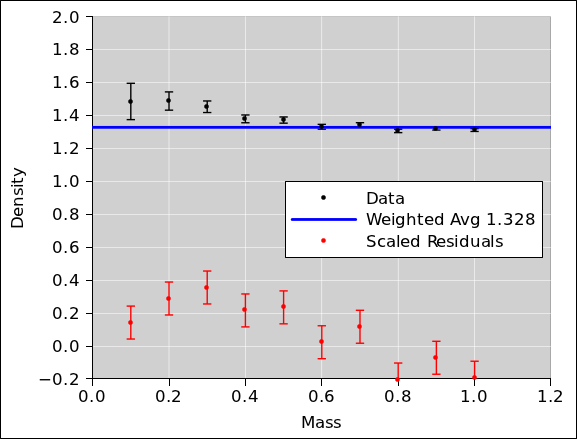Figure 12: Density versus Mass, with Residuals

Our suspicions are confirmed; there is a definite northwest/southeast trend to the residuals. This is not good. There is some kind of systematic problem.

As mentioned in section 3.3, sometimes people who ought to know better suggest that throwing in a superfluous fitting parameter is a good way to check for and/or correct for systematic errors. Applying this idea to our example gives the results shown in figure 13. As you can see, adding a parameter to the model without understanding the problem is somewhere between unprofessional and completely insane. It is not successful in detecting (let alone correcting) the problem.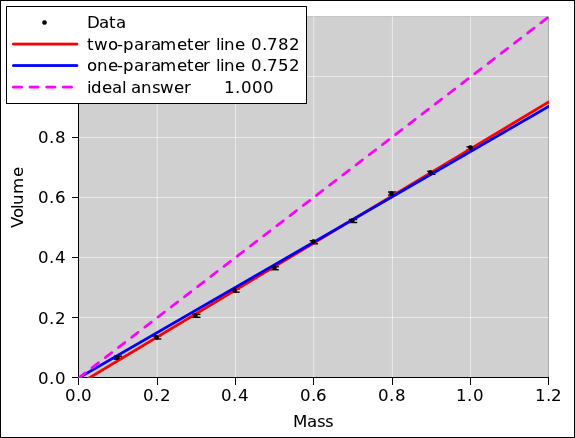Figure 13: Volume versus Mass, Fit with “Extra” Parameter

After thinking about the problem for a long time, we discover that the material is slightly porous. When we measure the volume by seeing how much water is displaced by the sample, the water percolates into the sample for a distance of 0.2 units. As a result, there is a “shell” surrounding a “core”. Specifically, it turns out that all along, the data was synthesized by stipulating that the effective displacement of the sample is 100% of the core volume plus 2/3rds of the shell volume, plus some noise.

Now that we understand what is going on, we can build a well-founded model. Specifically, we will fit to a two-parameter model, hoping to find the density of the core and the (apparent) density of the shell. The results are shown in figure 14.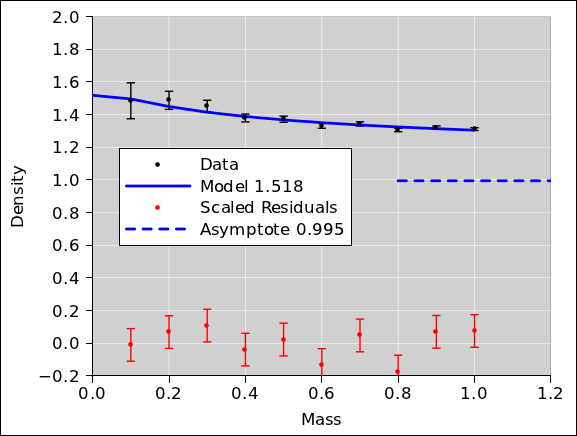Figure 14: Density versus Mass, Fancy Model

Note that the residuals are much better behaved now. They are closer to zero, and exhibit no particular trend.

As you can see from the y-intercept of the model, the apparent density of the shell is 1.518. This is very nearly the ideal answer i.e. 1.5 i.e. the reciprocal of 2/3rds.2

Meanwhile, the other fitted parameter tells us the asymptotic density, i.e. the density of the core, which is 0.995. This is very nearly the ideal answer i.e. 1.0. Note that this is incomparably more accurate than the result we would have gotten via simple one-parameter averaging (as in figure 12) or via a dumb two-parameter straight-line fit (as in figure 13). Even if you took data all the way out to volume=10 or volume=100 the shell would cause a significant systematic error. That is to say, the apparent density of the sample approaches the asymptotic density only slowly ... very very slowly.

You can see that in order to make an accurate determination of the density of the material, it was necessary to account for the systematic error. Random noise on the mass and volume measurements was nowhere near being the dominant contribution to the overall error.

• In this example, we chose to deal with the systematic error by building a model that accounts for what is going on. This is a tricky business, building something that is detailed enough to be a faithful model, yet not so complicated that it introduces too many additional adjustable parameters.
• Another approach would be to change the experimental method so that it is less sensitive to porosity. One could imagine using 3D laser scanning (rather than liquid displacement) to ascertain the volume. Alternatively, depending on the material, one could imagine sculpting it into an accurate spherical shape, measuring the diameter with calipers, and using that to infer the volume.

If the data had been less noisy, the importance of dealing with the systematic error would have been even more spectacular. (I dialed up the noise on the raw data to make the error bars in figure 9 more easily visible. The downside is that as a result, the systematic errors are only moderately spectacular. They don’t stand out from the noise by as many orders of magnitude as they otherwise would. It is instructive to reduce the noise and re-run the simulation.)

## 5  Principles of Linear Least-Squares Fitting

### 5.1  Basic Notions

In general, linear regression can be understood as follows. We want to find a fitted function that is a linear combination of certain basis functions.

To say the same thing in mathematical terms, we want to find a best-fit function B that takes the following form:

B(i) = a0 b0(i) + a1 b1(i) + a2 b2(i) + ⋯ + aM−1 bM−1(i)     (4a)
=
 ∑ j
aj bj(i
(4b)

The aj are called the coefficients, and the bj are called the basis functions. There are M coefficients, where M can be any integer from 1 on up. Naturally, there are also M basis functions.

Using vector notation, we can rewrite equation 4 as

 B(i) = a · b(i) (5a) = ⟨a | b(i)⟩ (5b)

where a is an M-dimensional vector, and where b is a vector-valued function of i. Equation 5b uses Dirac bra-ket notation to represent the dot product.

Given a set of observed Y-values {Y(i)} and a set of basis functions, the simplified fitting procedure finds the optimal coefficient-vector a| ... where “optimal” is defined in terms of minimizing the distance

Du =
 ∑ i
[Y(i) − B(i)]2
(6a)
=
 ∑ i
[Y(i) − a · b(i)]2
(6b)

where Du is the naïve unweighted distance. The weighted distance is:

D =
 ∑ i
wi [Y(i) − a · b(i)]2
 ∑ i
wi
(7)

When minimizing D, you can ignore the denominator in equation 7, since it is a constant.

It must be emphasized that the coefficients aj are always independent of i; that is, independent of which data point we are talking about. In contrast, the weights wi depend on i but are independent of j, i.e. independent of which basis function we are talking about.

### 5.2  Weighting

Pedagogical suggestion:

• When first introducing the topic, don’t even mention weights. Let all fits be unweighted, by which we mean equally-weighted. This applies to curve fitting in general, and to averaging in particular, which I consider to be just a particularly simple type of curve fitting.
• On the next turn of the pedagogical spiral, the motto should be:
• All averages are weighted averages.
• All fits are weighted fits.

In this section, we assume each data point has a nontrivial weight associated with it. If the data is uncorrelated, we should minimize the weighted distance:

D =
 ∑ i

 [Y(i) − a · b(i)]2 σ2(i)
(8)

where σ(i) is the uncertainty on the ith point, and the weighting factor W(i) ≡ 1/σ2(i) tells how much emphasis to give the ith point. Alas the spreadsheet linest(...) function does not allow you to specify the weights. It forces you to give all the points the same weight. This is, in general, a very bad practice. The workaround for this problem is to pull a factor of σ(i) out of every point in the data, and out of every point in every basis function. This is doable, but incredibly annoying. Among other things, it means the supposedly-constant function b0(i) in equation 11a is no longer constant, i.e. no longer independent of i. This means you must set the third argument of linest(y_vec, basis_vecs, affine) to zero, and then supply your own b0(i) function as one of the basis functions in the second argument.

More generally, we can handle correlated raw data as follows:

D =
 ∑ ij
[Y(i) − a · b(i)] wij [Y(j) − a · b(j)]
(9)

where w is the weight matrix.

Typical spreadsheet programs have a linest() function that doesn’t know anything about weights. For uncorrelated data, you can compensate for this easily enough, as follows: For each data point i, multiply the observed Y(i) and each of the basis functions b(i) by 1/σ. This produces the required factor of 1/σ2 in the objective function, in accordance with equation 8.

This is of course synonymous with multiplying the data and the objective functions by √(wii), in accordance with equation 9.

In the linest() function, you not enable the built-in feature that gives you a constant term. You need a column of constants to which weighting can be applied. Other than that, you do not need to allocate any new rows or columns, because you can do the division on the fly, dividing one array by another. If the observations are in column Y, the basic functions are in columns ABC, and the weights are in column W, you can use an expression like this:

```        =linest(Y1:Y10/W1:W10, A1:C10/W1:W10, 0, 1)
```

An example of this can be found on one of the sheets in reference 3.

### 5.3  Often, The Weights Come From the Model

Consider the data shown in figure 15.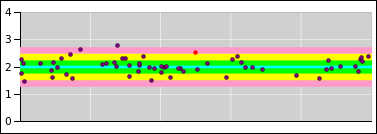Figure 15: Randomness = Property of the Distribution

You will notice that the data points to not have error bars associated with them. Instead I used the model to plot:

• a best-fit line (the central cyan line)
• a 1σ tolerance band (the green shaded region)
• a 2σ tolerance band (the yellow shaded region)
• a 3σ tolerance band (the pink shaded region)

The model (including the tolerance band) is plotted in the background, and then the zero-sized pointlike data points are plotted in the foreground.

I am not the only person to do things this way. You can look at some data from the search for the Higgs boson. The slide shows the 1σ (green) and 2σ (yellow) bands. The data points are pointlike. We get to ask whether the points are outside the band.

Reference 4 intro goes into more detail on this point, and shows additional ways way of visualizing the concept. Search for where it talks about “misbegotten error bars”. The point is, putting error bars on the raw data points is just wrong. It will give you the wrong fitted parameters. I am quite aware that typical data-analysis texts tell you to do it the wrong way.

Now the question arises, how do we achieve the desired state. In favorable cases, the weights can be determined directly from the abscissas. In least-squares fitting, the abscissas are considered known, so determining the weights is straightforward.

Sometimes, however, the weights depend on the model in more complicated ways. They may depend on the fitted parameters. This is a a chicken-and-egg problem, because the fitting procedure requires weights in order to do the fit, yet the fitted parameters are needed in order to calculate the weights.

The solution is to pick some initial estimate for the weights, and do an initial fit. The use the fitted parameters to obtain better weights, and iterate.

Let’s apply this to the example in reference 3. In order of decreasing desirability:

• You could measure the weight of the spring, and use theory to set the initial extra-mass parameter to 1/3rd of the mass of the spring. This is exact for an ideal spring. It is only approximate for real springs, but it’s good enough for an initial estimate.
• You could set the extra-mass parameter to zero, as a first approximation. The weights then depend only on the bob mass, which is known in advance.
• If you’re really lazy, you could use uniform weights as an initial guess.

Note that nonlinear least-squares fitting is always iterative. If you have to hunt for suitable weights, even linear least-squares fitting becomes iterative.

### 5.4  Background: What’s Linear and What’s Not

The documentation for linest(...) is confusing in at least two ways:

 The documentation claims the linest() function explains Y(i) in terms of what it calls “X(i)” but we are calling the basis functions bj(i). We write “X(i)” in scare quotes to avoid confusion. In fact, in terms of dimensional analysis, the basis functions don’t even have the same dimensions as x. Consider the case where y(x) is voltage as a function of time; the basis functions necessarily have dimensions of voltage, i.e. the same as y (not time, i.e. not the same as x). IMHO the linest() function should be documented as linest(y_vec, basis_vecs, ...) or something like that.   In contrast, the genuine x (without scare quotes) means something else entirely: Suppose you think of y as a function of genuine x, plotted on a graph with a y-axis and a x-axis. Then it is emphatically not necessary for this genuine x to be one of the basis functions bj. The poster child for this is a Fourier series (with no DC term), where the genuine x is not one of the basis functions.   Let’s be clear: the fitting procedure cares only about bj(i), that is, the value of the jth basis function associated with the ith data point. It does not care about the genuine x-value associated with the ith point, unless you choose to make x one of the basis functions. The x-axis need not even exist.   To say the same thing another way: Linear regression means that the best-fit function B needs to be a linear function of the parameters aj. It does not mean that the jth basis function bj(i) needs to be a linear function of i. Again, the poster child for this is a Fourier series (with no DC term), where none of the basis functions is linear.

Here’s another point of possible confusion:

 The documentation says you should set the third argument (affine) to TRUE if «the model contains a constant term». What it actually means is that third argument should be set to TRUE if (and only if) you want an x0 basis function to be thrown into the model in addition to whatever basis functions you specified explicitly in the second argument.   To say the same thing the other way, if you included an explicit x0 basis function in the second argument, the third argument needs to be FALSE.   In particular, if you are doing a weighted fit (as you almost certainly should be), you need to set the third argument (“affine”) to FALSE and provide your own x0 points, properly weighted. (There is no way to apply weights to the built-in “affine” term.)

### 5.5  Example: Fitting a Straight Line

Keep in mind the warning in section 5.4: The basis functions do not need to be linear. A lot of people are confused about this point, because the special case described by equation 11 is the most common case. That is to say, it is common to choose the genuine x-value to be one of the basis functions. Not necessary but common. We can express that mathematically as:

 b1(i) = I(x(i)) (the identity function) = x(i) (for all i)
(10)

where x(i) is the genuine x-value that you plot on the x-axis.

In particular, fitting a straight line with a two-parameter fit means choosing b1 to be the identity function (equation 10 or equation 11b), and choosing b0 to be the trivial constant function (equation 11a):

 b0(i) = 1 (for all i) (11a) b1(i) = x(i) (for all i) (11b)

This linear equation can be contrasted with the quadratic in equation 14.

A one-parameter fit is used when the straight line is constrained to go through the origin. It omits the constant basis function (equation 11a).

#### 5.5.1  Fitting to Points Within an Error Band

Figure 16 shows a straight line fitted to four data points. Because of the symmetry of the situation, the fitted line has zero slope.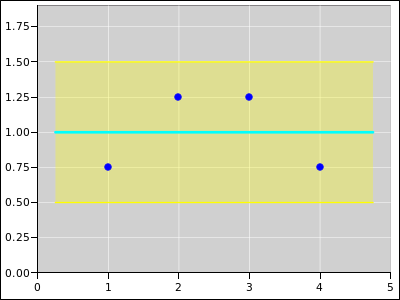Figure 16: Straight Line Fitted to 4 Points; Equally Weighted

Figure 17 shows the same situation, except that it is a weighted fit. Point #3 has four times the weight of any of the other points. The weight comes from the model. The model says that the error band is only half as wide at abscissa #3.Figure 17: Straight Line Fitted to 4 Points; Extra Weight on Point #3

Figure 18 shows the same situation, except that oint #4 is the one with the extra weight.Figure 18: Straight Line Fitted to 4 Points; Extra Weight on Point #4

#### 5.5.2  Fitting to Distributions with Error Bars

A lot of people are accustomed to thinking in terms of error bars. This is very often the wrong thing to do. When fitting to raw data points, such as the blue points in section 5.5.1, it is better to think in terms of zero-sized pointlike points, with no error bars, sitting within an error band.

However, sometimes you get information that is not in the form of raw data points, but rather cooked distributions. The width of the distribution can be represented by error bars. Let’s be clear: the blue plotting symbols in section 5.5.1 represent numbers, whereas the red plotting symbols in this section represent distributions. A number is different from a distribution as surely as a scalar is different from a high-dimensional vector.

The mathematics of weighted fitting is the same in this case, even though the interpretation of resulting fit is quite different. This is shown in the following diagrams.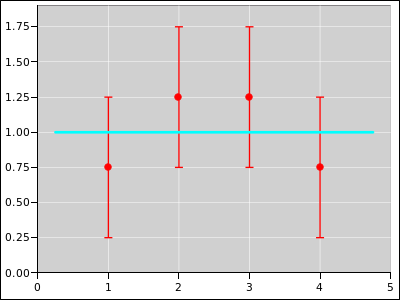Figure 19: Straight Line Fitted to 4 Distributions; Equally WeightedFigure 20: Straight Line Fitted to 4 Distributions; Extra Weight on #3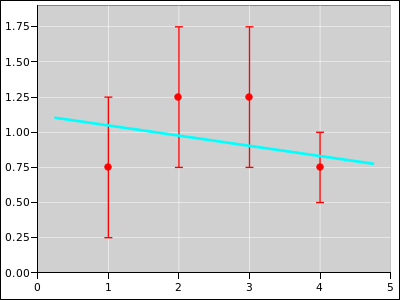Figure 21: Straight Line Fitted to 4 Distributions; Extra Weight on #4

### 5.6  Tactics: Weighted Linest

It is not difficult to perform weighted fits using the usual implementation of linest(), even though the documentation doesn’t say anything about it. The procedure is messy and arcane, but not difficult.

The first step is to not use the built-in “affine” feature. Instead create an explicit basis function with constant values, as in equation 11a. This basis function can be weighted, whereas the built-in “affine” feature cannot.

The next step is to create a copy of all your y-values and all your basis functions, scaled by the square root of the weight:

y′(i) =
y(i
 w(i)

bj(i) =
bj(i
 w(i)
for all j
(12)

Then apply the linest() function to the primed quantities.

Note that the weight of a point is inversely proportional to the uncertainty squared. So multiplying by the square root of the weight is the same as dividing by the uncertainty to the first power:

 y′(i) = y(i) / σ(i) b′j(i) = bj(i) / σ(i) for all j
(13)

The scaling factor applied by these equations affects both terms within the square brackets in equation 6b. It gets squared along with everything else, so the effect is the same as the weighting factor in the numerator of equation 7, as desired.

Keep in mind that linest() has no notion of continuity of y as a function of x. It treats the ith point (xi, yi) independently of all other points. Therefore scaling the y-values in accordance with equation ?? does no harm. It has no effect other than the desired weighting factor.

### 5.7  Another Example: Fitting a Quadratic

A quadratic is perhaps the simplest possible nonlinear function. Figure 22 shows an example of using multi-parameter least-squares fitting to fit a quadratic to data. The fit (and the figure) were produced using the "polynomial-plain" page of the spreadsheet in reference 5.In the figure, the blue points are the observed data. For the purposes of this example, the data was cobbled up using the ideal distribution given by the black curve, plus some noise. (In the real world, you do not normally have the ideal distribution available; all you are given are the observed data.) The magenta curve is the optimal3 quadratic fit to the data.

One slightly tricky thing about this example is the following: When entering the "linest" expression into cells Q5:S5, you have to enter it as an array expression. To do that, highlight all three of the cells, then type in the formula, and then hit <ctrl-shift-enter> (not simply <enter>). That is to say, while holding down the <ctrl> and <shift> keys with one hand, hit the <enter> key with the other hand.

More generally, whenever entering a formula that produces an array (rather than a single cell), you need to use <ctrl-shift-enter> (rather than simply <enter>). Another application of this rule applies to the transpose formula in cells O5:O7.

For details about what the linest() function does, see reference 6.

Also note the Box-Muller transform in columns A through D. This is the standard way to generate a normal Gaussian distribution of random numbers. Depending on what version of spreadsheet you are using, and what add-ons you have installed, there may be or may not be easier ways of generating Gaussian noise. I habitually do it this way, to maximize portability.

Note that if you hit the <F9> key, it will recalculate the spreadsheet using a new set of random numbers. This allows you to appreciate the variability in the results.

Note that if you look at the formula in cell Q5, the second argument to the linest() function explicitly specifies two basis functions, namely the functions4 in columns J and K. However, the coefficient-vector found by linest() and returned in cells Q5 through S5 is a vector with three (not two) components. That’s because by default, linest() implicitly uses the constant function (equation 11a) as one of the basis functions, in addition to whatever basis functions you explicitly specify. (If you don’t want this, you can turn it off using the optional third argument to linest(). An example of a fit that does not involve the constant function can be found in section 5.8.)

To summarize, the basis functions used for this quadratic fit are

 b0(i) = 1 (for all i) b1(i) = x(i) b2(i) = x2(i)
(14)

This can be considered an extension or elaboration of equation 11.

Last but not least, note that linest() returns the coefficients in reverse order! That is, in cells Q5, R5, and S5, we have the coefficients a2, a1, and a0 respectively. I cannot imagine any good reason for this, but it is what it is.

### 5.8  Fancier Example: Fitting a Fourier Series

For our next example, we fit some data to a three-term Fourier sine series. That is to say, we choose to use the following three basis functions:

 b0(i) = sin(1 (2π) xi) b1(i) = sin(2 (2π) xi) b2(i) = sin(3 (2π) xi)
(15)

As a premise of this scenario, we assume a priori that we are looking for an odd function. Therefore we do not include any cosines in the basis set. This also means that we do not include the constant function (equation 11a) in the basis set. The constant function can be considered a zero-frequency cosine, so there is really only one assumption here, not two. We need to be careful about this, because the linest(...) function will stick in the constant function unless we explicitly tell it not to, by specifying false as the third argument to linest(...), as you can see in cell I2 of the Fourier-triangle tab in reference 5.

The color code in figure 23 is the same as in figure 22. That is, the blue points are the data. For the purposes of this example, the data was cobbled up using the ideal distribution given by the black curve, plus some noise. The magenta curve is the optimal5 quadratic fit to the data.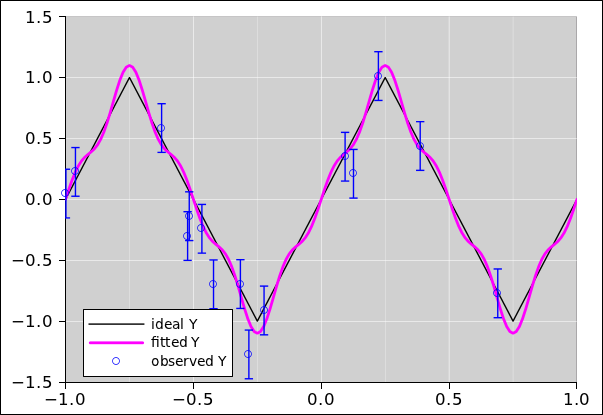Figure 23: Fitting a Fourier Series to Triangle-Wave Data

As implemented in reference 5, in cells I24 through K38 we tablulate the basis functions. However, this is just for show, and these tabulated values are not used by the linest(...) function. You could delete these cells and it would have no effect on the calculation. Instead, the linest(...) function evaluates the basis functions on-the-fly, using the array constant trick as discussed in reference 7.

As always, the linest(...) function returns the fitted coefficients in reverse order. In contrast, we really prefer to have the coefficients in the correct order, as given in cells I9 through K9, so that they line up with the corresponding basis functions in cells I24 through K38. Alas there is not, so far as I know, any convenient spreadsheet function that returns the reverse of a list, but the reverse can be computed using the index(...) function, as you can see in cell I9.

The linest(...) function can also return information about the residuals and the overall quality of the fit. To enable this feature, you need to set the fourth argument of linest(...) to true. You also need to arrange for the results of the linest(...) to fill a block M columns wide and 5 rows high, where M is the number of basis functions; for example, M=2 for a two-parameter straight-line fit. That is, highlight a block of the right size, type in the formula, and then hit <ctrl-shift-enter>.

## 6  Uncertainty

We need to estimate the uncertainty associated with the fitted parameters. The procedures for doing this in the linear case are almost identical to the nonlinear case. See reference 8.

## 7  References

1.
John Denker,
./systematic-error-peculiar.xls

2.
John Denker, “Twenty Questions”
www.av8n.com/physics/twenty-questions.htm

3.
John Denker,
“spreadsheet for measuring spring constant by observing period”
./measure-k-oscillator.xls

4.
John Denker,
“Introduction to Probability”
www.av8n.com/physics/probability-intro.htm

5.
John Denker,
“spreadsheet demonstrating multi-parameter least-squares fitting using nonlinear basis functions”
./linear-regression.xls

6.
The Gnumeric Manual : LINEST
http://projects.gnome.org/gnumeric/doc/gnumeric-function-LINEST.shtml

7.
John Denker,

8.
John Denker,
“Nonlinear Least Squares”
www.av8n.com/physics/nonlinear-least-squares.htm

1
William of Ockham is famous for having made heavy use of it.
2
In this pedagogical example, we know the ideal “correct” answer because we are fitting to data that was cooked up by hand. In contrast, in the real world you almost never know the “correct” answer in advance. The whole point of performing the fit is to find the answer.
3
This “optimal” fit is optimal in the least-squares sense. This corresponds to maximum likelihood, i.e. maximum a priori probability. This is not necessarily optimal for any practical purpose. In many cases you would be better off with a maximum a posteriori probability. However, that is beyond the scope of the present discussion.
4
These are functions, because they are functions of row number. The row number plays almost the same role as the variable (i) in equation 5.
5
Again, beware that “optimal” in the least-squares sense is very commonly not what you really want.
[Contents]